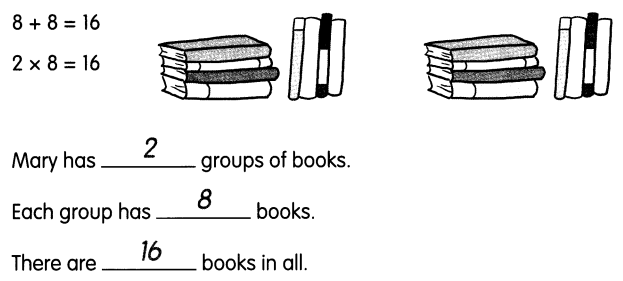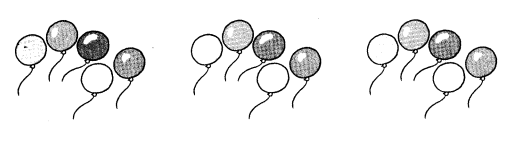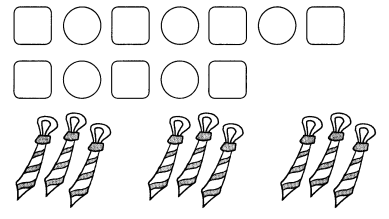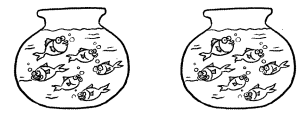# Math in Focus Grade 2 Chapter 5 Practice 2 Answer Key How to Multiply

Go through the Math in Focus Grade 2 Workbook Answer Key Chapter 5 Practice 2 How to Multiply to finish your assignments.

## Math in Focus Grade 2 Chapter 5 Practice 2 Answer Key How to Multiply

Look at the addition and multiplication sentences. Fill in the blanks.

ExampleQuestion 1.
5 + 5 + 5 = 15
3 × 5 = 15Patrick has ___ groups of balloons.
Each group has ________ balloons.
There are ________ balloons in all.
Patrick has 3 groups of balloons
Each group has 5 balloons
There are 15 balloons in all.

Write the addition and multiplication sentences. Fill in the blanks.

Question 2.Marcus has ___ groups of ties.
Each group has _________ ties.
There are _________ ties in all.
Given that number of ties are 9.
The 9 ties are divided into 3 groups.
Each group has 3 ties.
3 + 3 + 3 = 9
9 ÷ 3 = 3
Marcus has 3 groups of ties.
Each group has 3 ties.
There are 9 ties in all.

Fill in the blanks.

Question 3.How many bowls are there? _______
How many fish are ¡n each bowl? ___
How many fish are there in all?
_____ × _____ = _____
There are _________ fish in all.